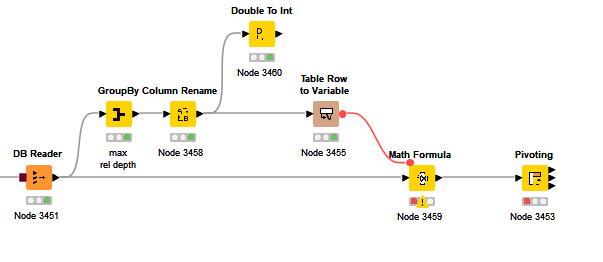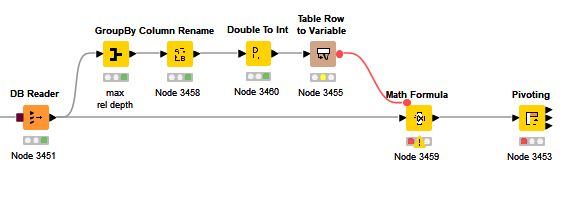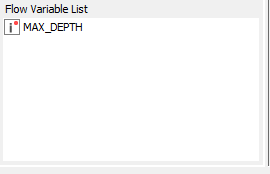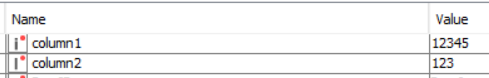# “Table Row To Variable” variables not accessible - BUG

Reopening “Table row to variable” variables not accessible as I get into another similar problem.
I have a longint in my DB that I need to agregate and then reinject in a Column Expressions node or Math Formula node.
Therefore I am using a Table Row To Variable node.
At the output of that node, I get a variable with the correct value, although it is of the Integer type.
When I try to inject in the Column Expression node (same behaviour with Math Formula), the variable is not visible.It is only visible when I convert the value to an integer before the Table Row To Variable node that I can see it. I am using a double to int node for this purpose (which was not obvious considering the node name BTWWhy does the Table Row to Variable node convert Longints into Integers, that are not actually Integers?
Is there a way to use longints flow variables as inputs to the Column Expressions node?

If you look closely, you can see that the Table Row to Variable node properly converts the long Column to a long Flow Variable, but the letters `i` and `l` are difficult to distinguish.